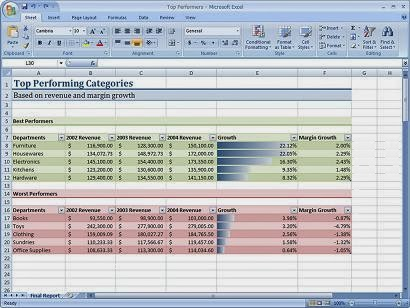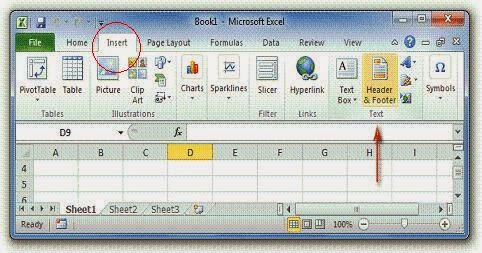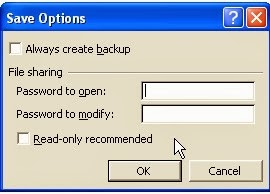# Explain Different Features of Microsoft Excel

•
•
•
•
•
•
•
•
•
•
Microsoft Excel is a spreadsheet software developed by Microsoft Corporation of USA. Microsoft Excel is used to input and format data and to apply suitable mathematical and other formulas on the data. Excel can perform large number of calculations on data easily in a table like format. The main file of Microsoft Excel is called an Excel Workbook. By default, each workbook has three Excel Worksheets. A Worksheet is divided into Rows, Columns and cells. Microsoft Excel provides many easy to use features as follows:

### Important Features Provided by MS Exceldifferent features of Microsoft Excel application software

### 1.    Auto Fill

MS Excel provides easy series generating feature called Auto Fill. With the help of Auto Fill you can fill the adjacent cell range with a series like days of a weak or a number series like 1,2,3,4,…10 or 5,10,15,…50 or 1990, 1991, 1992… or dates etc.Header and footer feature is used to display same text on top and bottom of each page. For example the information like page numbers, date written, author name, chapter name etc. can be written in header or footer.### 3.    Easy Formatting

The text and numeric data can be easily formatted in Microsoft Excel. For example, the font style, font size, font color and text alignment in cells can be easily changed.### 4.    Spelling and Grammar Check

Spelling and Grammar feature is used to help you to correct spelling and grammar errors. Spelling errors are underlined by red wavy line and the errors in Grammar are highlighted by green wavy line.Misspelled words can be correct by just clicking right button of mouse on the word, a menu with spelling suggestions will appear, select the suitable word, as shown in the figure above.

### 5.    Auto Correct

Auto Correct feature is used to correct many common misspelled words and punctuation marks.

### 6.    The Formula Bar

It is used to enter different formulas for calculations. For example, if we are to add three numbers then we will type the formula “=A1+A2+A3” in the cell of worksheet.### 7.    Built-in Functions

Excel has many built-in functions. You can use functions to apply lengthy calculations easily.For example, if we have to calculate total of first then cells from A1 to A10 then the formula is:
= A1+A2+A3+A4+A5+A6+A7+A8+A9+A10
This formula may be very very long if we are to take total of A1 to A100 hundred cells. Therefore we use built in functions like SUM function. We will type “=SUM(A1:A100)” in the cell and get the resultant total.### 8.     Sorting

Sorting feature is used to sort data in ascending or descending order. For Example we will sort Roll Number column in ascending order from 1,2,3 … and so on. On the other hand, we may chose to sort the percentage of marks obtained by students in Descending order to prepare a Merit List.

### 9.    Charts

Excel provides chart feature to present data in the form of charts and graphs for easy and graphical representation. In Microsoft Excel 2007 different types of charts can be created for numerical data stored in worksheets. Charts make it easy to compare and understand large amounts of numerical data. For example, consider the worksheet of a cricket match. If we record scores of the 11 batsmen of Cricket Team A as follows:

 S.No. Batsman Score 1 Inzamam 100 2 Afridi 50 3 Misbah 30 4 Shehzad 90 5 Nasir 2 6 Fahad 8 7 Kamran 35 8 Umer 25 9 Umer Gul 0 10 Saeed 12 11 Junaid 4

Note that we have to read whole table of names and scores if we want to find the highest or lowest scores by the batsmen. But looking at the chart created in Microsoft Excel, we can see the highest score by the highest column which is 100 scores of Inzamam.### 10.    Filter

Filter feature is used to see the required data according to some given criteria. In this way, we can see the needed data only, out of large amounts of data in worksheet. For Example we can apply top ten records filter to see only top ten students with highest marks in descending order.### 11.    Auto Complete

Auto Complete feature is used to automatically complete the word you are typing.For example, if you have already written a word say “Examination” in a cell of your worksheet, when you start typing this word again in another cell, the Auto Complete Feature of excel will show and fill the word “Examination” automatically in new cell.

You can apply security on Excel file by applying password. So that only authorized people (the people who have password) can open file.### 13.    PrintingYou can easily print excel files for hard copy. You can print worksheets in the form of separate printed pages. Normally MS Excel is used to prepare proformas of various kinds. These proformas are filled with required data and then print command is applied to get the proformas printed as hard copies.### 14.    Recalculation

Recalculation feature means the calculations in worksheet will be automatically recalculated if you change data in worksheet. For example, let we type 10 in cell A1, 20 in cell A2 and 30 in cell A3. Now we apply Sum function in cell A4 as “=Sum(A1:A3)” and press Enter key. The answer = 10+20+30 = 60 will be displayed in A4 cell. Now if we change data in cell A1 to zero. Then the total in cell A4 will be changed to 50 instantly. Because 0+20+30 will be recalculated automatically and answer 50 will be displayed in cell A4.### 15.    Email worksheets

In Excel you can send worksheets by Email to one or more persons. We can send prepared worksheets in MS Excel to send by Email when computer is connected to internet.

### 16. Pivot Tables

A pivot table may be defined as an interactive table that is used to quickly combine and compare large amounts of data. Pivot table is an excellent feature of MS Excel especially for business organizations to summarize important data quickly and easily.